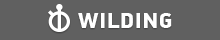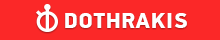GMAT Question of the Day - Daily to your Mailbox; hard ones only

 It is currently 26 Jun 2019, 11:17GMAT Club Daily Prep

Thank you for using the timer - this advanced tool can estimate your performance and suggest more practice questions. We have subscribed you to Daily Prep Questions via email.

Customized
for You

we will pick new questions that match your level based on your Timer History

Track

every week, we’ll send you an estimated GMAT score based on your performance

Practice
Pays

we will pick new questions that match your level based on your Timer History

Not interested in getting valuable practice questions and articles delivered to your email? No problem, unsubscribe here.If 3x + y = 14, and x and y are positive integers, each of the followi

Author Message
TAGS:

Hide Tags

Math ExpertV
Joined: 02 Sep 2009
Posts: 55803
If 3x + y = 14, and x and y are positive integers, each of the followi  [#permalink]

Show Tags00:00

Difficulty:25% (medium)

Question Stats:74% (01:45) correct26% (01:56) wrongbased on 45 sessions

HideShow timer StatisticsIf 3x + y = 14, and x and y are positive integers, each of the following could be the value of x + y EXCEPT

A. 12
B. 10
C. 8
D. 6
E. 4

_________________
Senior PS ModeratorD
Status: It always seems impossible until it's done.
Joined: 16 Sep 2016
Posts: 749
GMAT 1: 740 Q50 V40GMAT 2: 770 Q51 V42Re: If 3x + y = 14, and x and y are positive integers, each of the followi  [#permalink]

Show Tags

We know that x and y are positive integers so minimum value they can take is 1.

Also,
$$3x + y = 14$$
Giving y the minimum value of 1... it turns out x will not be an integer

Hence minimum value of y possible is 2
$$3x + 2 = 14$$
$$3x = 12$$
$$x = 4$$

So minimum value of x + y is 4 + 2 = 6

Anything lower than this is not possible - So Option (E) is the first one out.

Also, we know only one of the options can be correct. Therefore we can afford to not check any of the other options and go with (E).
Bunuel wrote:
If 3x + y = 14, and x and y are positive integers, each of the following could be the value of x + y EXCEPT

A. 12
B. 10
C. 8
D. 6
E. 4

_________________
Regards,

“Do. Or do not. There is no try.” - Yoda (The Empire Strikes Back)
ManagerB
Joined: 30 Oct 2018
Posts: 61
Re: If 3x + y = 14, and x and y are positive integers, each of the followi  [#permalink]

Show Tags

option A-> x=1, y=11
option B-> x=2, y=8
option C-> x=3, y=5
option D-> x=4, y=2

hence option E is not possible and is the answer.
_________________

----------------------------------
Plz Kudos! if my post helps....
CEOP
Joined: 18 Aug 2017
Posts: 3947
Location: India
Concentration: Sustainability, Marketing
GPA: 4
WE: Marketing (Energy and Utilities)
Re: If 3x + y = 14, and x and y are positive integers, each of the followi  [#permalink]

Show Tags

Bunuel wrote:
If 3x + y = 14, and x and y are positive integers, each of the following could be the value of x + y EXCEPT

A. 12
B. 10
C. 8
D. 6
E. 4

3x+y=14
would stand true
@x=1,2,3,4 where y = 11,8,5,2
x+y= 12,10,8,6
IMO E ;4 stands out
_________________
If you liked my solution then please give Kudos. Kudos encourage active discussions.Re: If 3x + y = 14, and x and y are positive integers, each of the followi   [#permalink] 11 Jan 2019, 02:39
Display posts from previous: Sort by

If 3x + y = 14, and x and y are positive integers, each of the followi